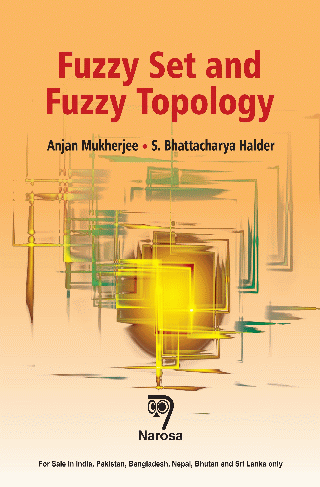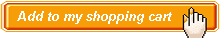Fuzzy Set and Fuzzy Topology Authors:   Anjan Mukherjee, Sharmistha Bhattacharya Halder ISBN: 978-81-8487-372-6 Publication Year:   2015 Pages:   188 Binding:   Paper Back About the book FUZZY SET AND FUZZY TOPOLOGY focuses in the various fields of fuzzy set and fuzzy topology. The concepts of fuzzy set has been introduced by Lotfi A. Zadeh in 1965. Later on lots of Researchers have worked in this field and its applications. K.T. Atanossov introduced the concept of Intuitionistic fuzzy topology. In this book beginning from the very basic concepts of fuzzy set, fuzzy relations, fuzzy graph, fuzzy topology, generalized fuzzy topology etc. are introduced and discussed in details. Key Features Table of content Preface / List of Symbols / Fuzzy Set Theory / Types of Fuzzy Sets / Fuzzy Relations and Fuzzy Graphs / Fuzzy Topological Spaces / Induced Fuzzy Topological Spaces / Connectedness in Fuzzy Topological Space / Separation Axioms / Fuzzy Compactness / Various Operators in Fuzzy Topological Space / References / Index.Audience Postgraduate Students, Professionals and Researchers